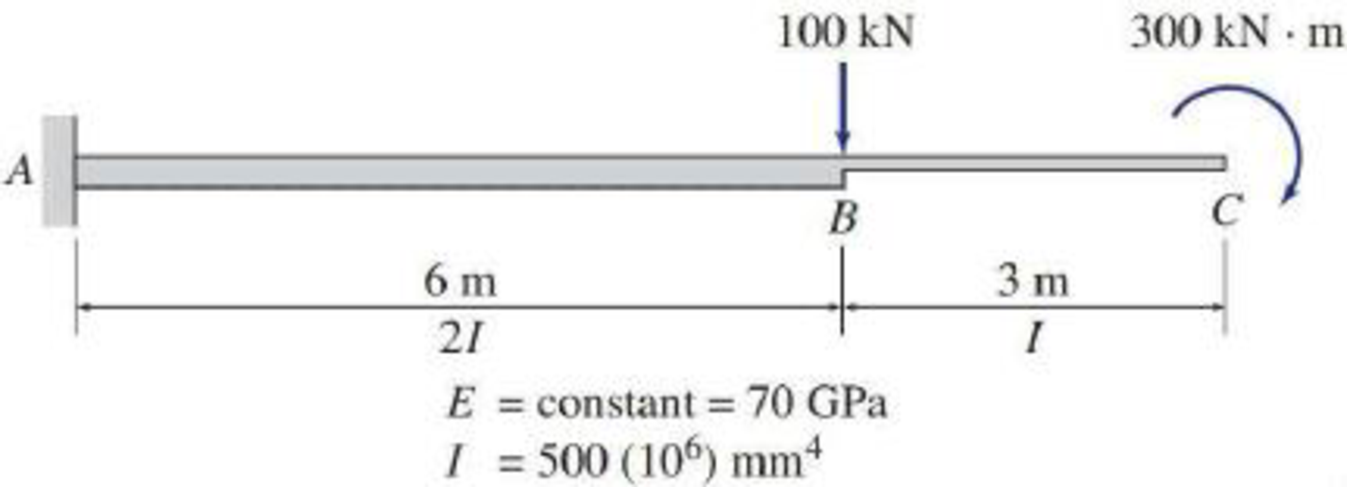# 6.14 through 6.17 Use the moment-area method to determine the slopes and deflections at points B and C of the beam shown. FIG. P6.15, P6.41

#### Solutions

Chapter
Section
Chapter 6, Problem 15P
Textbook Problem
1481 views

## 6.14 through 6.17 Use the moment-area method to determine the slopes and deflections at points B and C of the beam shown.FIG. P6.15, P6.41To determine

Find the slope θB&θC and deflection ΔB&ΔC at point B and C of the given beam using the moment-area method.

### Explanation of Solution

Given information:

The Young’s modulus (E) is 70 GPa.

The moment of inertia (I) is 500×106mm4.

Calculation:

Consider elastic modulus E of the beam is constant.

Show the given beam as in Figure (1).

Refer Figure (1),

Since the point C is free end there is no support reaction at Point C. Therefore, the reaction at point C is also equal to zero.

Consider upward is positive and downward is negative.

Consider clockwise is negative and counterclockwise is positive.

Determine the reaction at support A using the Equation of equilibrium;

V=0RA+RC100=0RA+0100=0RA=100kN

Determine the bending moment at C;

MC=300kNm

Determine the bending moment at B;

MB=300kNm

Determine the bending moment at A;

MA=100×6+300=900kNm

Show the M/EI diagram for the given beam as in Figure (2).

Elastic curve:

The sign of M/EI diagram is negative, therefore, the beam bends downward. The support A of the given beam is fixed and the slope at A is zero. Therefore, the tangent to the elastic curve at A is horizontal.

Show the elastic curve diagram as in Figure (3).

The slope at point B can be calculated by evaluating the change in slope between A and B.

Express the change in slope using the first moment-area theorem as follows:

θB=θBA=AreaoftheM/EIbetweenAandB=Areaoftriangle+Areaofrectangle=12×b×h1+(b×h2)

Here, b is the width, h1 is the height of the triangle, and h2 is the height of the rectangle.

Substitute 6 m for b, 300EI for h1, and 150EI for h2.

θB=θBA=(12×300EI(6)+150EI(6))=1EI(1,800)=1,800kNm2EI

Determine the slope at B using the relation;

θB=1,800kNm2EI

Substitute 70 GPa for E and 500×106mm4 for I.

Hence, the slope at point B is 0.0514rad(Clockwise)_.

The deflection of B with respect to the undeforemd axis of the beam is equal to the tangential deviation of B from the tangent at A.

Express the deflection at B using the second moment-area theorem as follows:

Here, b is the width of triangle and rectangle, h1 is the height of triangle, and h2 is the height of rectangle.

Substitute 6 for b, 300EI for h1, and 150EI for h2.

ΔB=ΔBA=(12×300EI(6)(23×6)+150EI(6)×62)=6,300kNm3EI

Determine the deflection at B using the relation;

ΔB=ΔBA=6,300kNm3EI

Substitute 70 GPa for E and 500×106mm4 for I.

ΔB=ΔBA=6,300kNm3(70GPa×106kN/m21GPa)(500×106mm4×(1m1,000mm)4)=0

### Still sussing out bartleby?

Check out a sample textbook solution.

See a sample solution

#### The Solution to Your Study Problems

Bartleby provides explanations to thousands of textbook problems written by our experts, many with advanced degrees!

Get Started

Find more solutions based on key concepts
Obtain a graduated beaker and accurate scale from a chemistry lab and measure the density of the following liqu...

Engineering Fundamentals: An Introduction to Engineering (MindTap Course List)

Discuss the difference between discrete and process manufacturing.

Principles of Information Systems (MindTap Course List)

Why are entity integrity and referential integrity important in a database?

Database Systems: Design, Implementation, & Management

What is an ATC?

Precision Machining Technology (MindTap Course List)

What is an effective way to assess user requests for additional features and functions?

Systems Analysis and Design (Shelly Cashman Series) (MindTap Course List)

The resultant of the 50-Ib and 30-lb forces is R. If R = 65 lb, determine the angles and .

International Edition---engineering Mechanics: Statics, 4th Edition

What is a transaction log, and what is its function?

Database Systems: Design, Implementation, & Management

What additional requirements are associated with a dedicated server? (116)

Enhanced Discovering Computers 2017 (Shelly Cashman Series) (MindTap Course List)

If your motherboard supports ECC DDR3 memory, can you substitute non-ECC DDR3 memory?

A+ Guide to Hardware (Standalone Book) (MindTap Course List)

What types of injuries can occur to the ears during welding?

Welding: Principles and Applications (MindTap Course List)